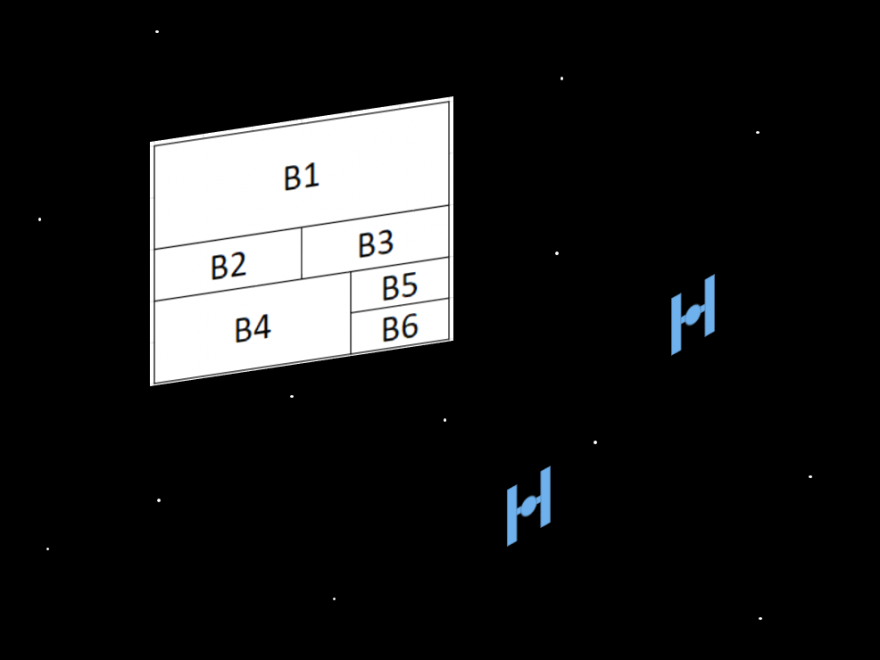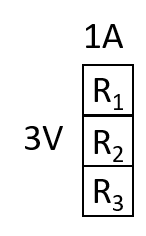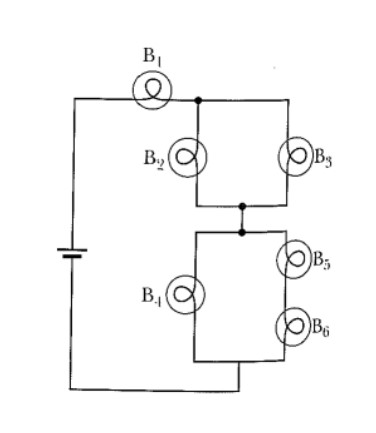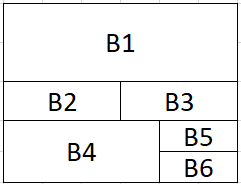That’s no schematic, it’s a power box

Title: Power Boxes: New Representation for Analyzing DC Circuits
Authors: Daryl McPadden, Jason Dowd, and Eric Brewe
First author’s institution: Michigan State University and Florida International University
Journal: The Physics Teacher 56, 362 (2018) (Closed access)

Typically electrical circuits are taught using schematics, which are simplified diagrams of electrical circuits. However, students often have difficulties reasoning qualitatively using these type of diagrams. Today’s paper introduces a new type of representation, the power box. Using a power box allows students to track changes in the voltage and current around a circuit and see how the power is distributed between various components. From this representation, students can easily distinguish between elements in series (they share the same current) and elements in parallel (they share the same voltage) and apply Kirchhoff’s loop and node rules. In today’s post, we provide an overview of the power box representation and show how it can be used to solve problems that would be difficult for a typical introductory physics student.

Creating a Tracking Power Box

In their paper, the authors describe two types of power boxes, a tracking power box and a summary power box. A tracking power box shows the current and voltage at a certain point in the circuit. Let’s start with the sample series circuit in figure 1. The circuit is a series circuit since there is only one path that does not retrace itself from the positive side of the battery to the negative side of the battery. (denoted by two parallel lines where the longer line represents the positive terminal of the battery).

To construct a power box, we start by finding the voltage and the current through the circuit. The battery has a voltage of 3V so the voltage across the circuit is 3V. If we assume each resistor has a resistance of 1Ω, the total resistance is 3Ω (the total resistance is the sum of the individual resistances for a series circuit). From Ohm’s law, the voltage is the resistance times the current, the total current is 1A. We can now draw a power box at point A. We start by drawing a box with the height of the voltage (3V) and a width equal to that of the current (1A). As the voltage drops across each element of the circuit, we leave a portion of the box corresponding to the amount of voltage dropped unshaded. Since we have not crossed the first resistor, there is no voltage drop so the power box will still be fully shaded (figure 2).Figure 2: Series circuit with power box at point A.

Now when we cross the first resistor, the voltage decreases by V=IR so 1A * 1Ω=1V. Therefore, we leave an area of the power box that is 1V high by 1A wide unshaded (figure 3).Figure 3: Series circuit with power box at point B. The voltage dropped by 1V and the current is unchanged so an area of 1V by 1A is unshaded.

Since each resistor has the same resistance and current through it, each resistor will have the same voltage drop. After crossing each resistor, an additional area of 1V by 1A will become unshaded (figure 4).Figure 4: Series circuit with power box now at point C.

After the third resistor, the voltage with respect to the negative side of the battery is 0 but there is still current. We represent this at point E with a solid black line (figure 5).Figure 5: Series circuit with power box added at point D. This figure now shows the tracking power boxes at each relevant point in the circuit. Note there is a thick black line at the bottom of the power box at point D to show there is still current.

Now we have it, our first tracking power boxes. The tracking label comes from the fact that we have tracked the power (the area of the boxes) around the circuit.

Repeating With a Parallel Circuit

Now let’s try to make tracking power boxes for the circuit shown in figure 6.

Since there are multiple non-backtracking paths to get from the positive terminal of the battery to the negative terminal of the battery, this is a parallel circuit. This means that each resistor will have the same voltage across it. The battery is 3V so that means each resistor will have 3V across it. Using Ohm’s law (V=IR), each resistor will have a current of 3A through it. That means overall, the current is 9A. Now let’s make the power boxes!

We start by drawing a box that is 3V high and 9A wide at point A (figure 7).Figure 7: The sample parallel circuit with a power box at point A.

As we have not crossed any of the resistors, the box will be fully shaded. When we get to point B, the current is now 3A but the voltage is still 3V. We then draw a vertical dotted line to represent this. When we get to point E, the voltage has dropped by 3V so we leave an area that is 3V by 3A unshaded (figure 8). Since each resistor has the same resistance, we do the same thing for points C, D, F, and G (figure 9).Figure 8: Parallel circuit with power boxes at points B and E.Figure 9: Parallel circuit with power boxes at point C, D, F, G, and H.

When we get to point H, the current is again 9A so we draw a thick horizontal line across the entire bottom of the box. And there we have it, another set of tracking power boxes to describe the circuit.

Summary Power Boxes

Now that we’ve created tracking power boxes, we can create a summary power box which shows how the power is distributed in the circuit. For example. using the tracking power boxes we created for the series circuit, we can show how much each resistor consumes of the total power (figure 10). Since each box has the same area, each resistor is consuming the same amount of power. Not only can we see the power each resistor consumes, we can see the layout of the circuit. Each resistor has the same current which means they all must be in series with each other. The general rule is that if two elements are in series, there is a horizontal line dividing them (because they have the same current) and if two elements are in parallel, a vertical line divides them (because they have the same voltage).Figure 10: A summary power box for the circuit shown in figure 1.

A Real Example

So why would anyone actually use this representation? Let’s go to a more challenging circuit (pulled from notes of a university physics course, figure 11).Figure 11: A sample complex circuit that a student could be asked to analyze as part of a homework or exam problem.

A fairly typical question to ask students is to determine what happens to the brightness of one bulb if a different bulb burns out, for example what happens to the brightness of B2 if B3 burns out (and hence can be ignored in the circuit). Let’s make a power box to do this.

We’ll do this part qualitatively to avoid too many calculations. Simply based on which bulbs are in series and which are in parallel, the power box has to look something like this (figure 12).Figure 12: Qualitative summary power box for the circuit shown in figure 11.

Now the question is very straightforward. If B3 is removed, the only way to conserve energy (and keep the power box a rectangle) is to increase the width of B2 since all the boxes must be rectangular. Therefore, since the area of B2 increased, B2 gets brighter. There was one thing we overlooked however. Since B3 is removed and was in parallel, the overall resistance of the circuit increases and the current decreases. Therefore, the summary power box should become less wide. Since the overall current decreases, the power box’s width will scale according and B2 will still need to become wider to “fill the gap” meaning that it becomes brighter.

We can do the same type of analysis with the other bulbs. If B1 was removed, all the bulbs should get brighter as there will need to be a greater voltage drop across each bulb for the total voltage drop to be equal to that of the battery. In the power box, this is equivalent to having all of the other rectangles increase in height to “fill the gap.”

Further, if the student were asked to find the current through or the voltage across one of the bulbs during Kirchhoff’s laws, the equations can easily be obtained with the power box. For example, the width of boxes B2 and B3 have the same width as B1. Since width is current, we have I1=I2+I3. Likewise, I1=I4+I5 since B4 and B5 stretch the width of the box. We can keep doing this to relate all of the currents and these relationships are the Kirchhoff current rules.

We can do the same thing with the voltages. If the battery has a voltage VB, we must have VB=V1+V2+V4 since B1, B2, and B4 span the height of the power box. Alternatively, we could use VB=V1+V3+V5+V6. Again, all the possible relationships we can make from the power box correspond to Kirchhoff’s voltage laws.

Some Limitations

While the power box is a useful representation, there are some limitations. First, the power box cannot show negative voltages, which requires the reference voltage to be the negative terminal of the battery. For the circuits used here, that isn’t a problem but for multi-battery circuits, this can become a problem. In addition, power boxes are not very useful for circuits with time dependent elements such as capacitors and inductors. These elements change the overall current and voltage in the circuit over time and hence the size of the power box will change. Therefore, power boxes work best with time independent elements or at fixed times.

Takeaways

Power boxes are a new representation that act as an intermediary between schematics and algebraic equations for analyzing circuits. Using this representation, students can visually compare the power used by an element of the circuit as well as see the voltage across and current through the element. In addition, power boxes allow students to easily determine which elements are in parallel and which are in series. So, next time circuits come up in class, why not include power boxes as part of the lesson?

For an interactive power box app, check out this one created by Andrew Duffy.